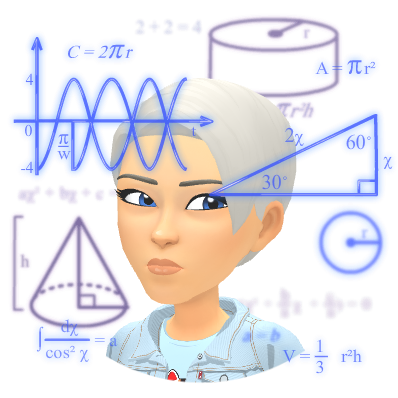Math - North Carolina Common Core

In 4t grade, mathematics instruction is focused on three critical areas:

1. Developing understanding and fluency in multi-digit multiplication, and developing understanding of dividing to find quotients involving multi-digit dividends.
2. Developing an understanding of fraction equivalences, addition and subtraction of fractions with like denominators, and multiplication of fractions by whole numbers.
3. Understanding that geometric figures can be analyzed and classified based on their properties, such as having parallel sides, perpendicular sides their angle measurement, and symmetry.
Helpful instructive videos can be found at learnzillion.com!

Operations and Algebraic Thinking
• Use the four operations with whole numbers to solve problems.
• Gain familiarity with factors and multiples.
• Generate and analyze patterns.
Number and Operations in Base Ten• Generalize place value understanding for multi-digit whole numbers.
• Use place value understanding and properties of operations to perform multi-digit arithmetic.

Number and Operations—Fractions

• Extend understanding of fraction equivalence and ordering.
• Build fractions from unit fractions by applying and extending previous understandings of operations on whole numbers.
• Understand decimal notation for fractions, and compare decimal fractions.

Measurement and Data

• Solve problems involving measurement and conversion of measurements from a larger unit to a smaller unit.
• Represent and interpret data.
• Geometric measurement: understand concepts of angle and measure angles.

Geometry

• Draw and identify lines and angles, and classify shapes by properties of their lines and angles.

Mathematical Practices

1. Make sense of problems and persevere in solving them.
2. Reason abstractly and quantitatively.
3. Construct viable arguments and critique the reasoning of others.
4. Model with mathematics.
5. Use appropriate tools strategically.
6. Attend to precision.
7. Look for and make use of structure.
8. Look for and express regularity in repeated reasoning.